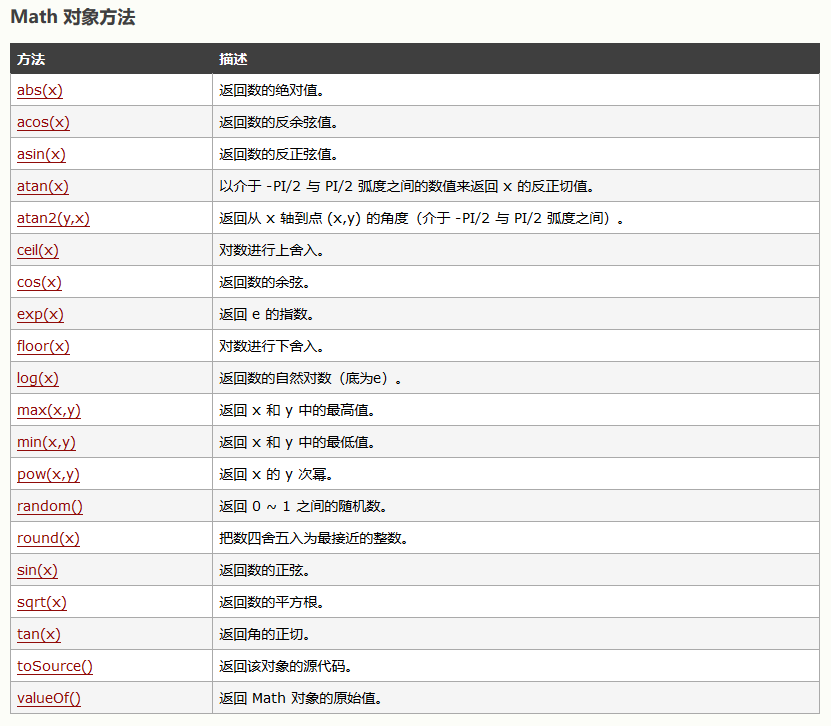• 下面小编就为大家带来一篇JavaScript Math 对象常用方法总结。小编觉得挺不错的，现在分享给大家，也给大家做个参考
• 首先Math对象是什么，Math对象的作用又是什么？ Math对象属于JavaScript对象中的内置对象，它不同于Array、Function、Object等对象可以创建构造，它是由ECMAScript实现提供，在程序开始执行时出现，可以通过调用其中...
• 主要介绍了JS Math对象与Math方法,结合实例形式总结分析了javascript中math对象常用属性与方法相关使用技巧,需要的朋友可以参考下
• 1.Math对象的PI属性，作用返回3.14圆周率 var PI=Math.PI console.log(PI)//打印输出 3.141592653589793 2.Math对象的abs()方法，作用返回一个绝对值 //各种数据类型的绝对值 var dou=Math.abs(-21.121) var ...
1.Math对象的PI属性，作用返回3.14圆周率
	var PI=Math.PI
console.log(PI)//打印输出 3.141592653589793

2.Math对象的abs()方法，作用返回一个绝对值
	//各种数据类型的绝对值

var dou=Math.abs(-21.121)
var num=Math.abs(2131)
var nul=Math.abs(null)
var nu=Math.abs(2*4)
var str=Math.abs("test")

console.log(dou)  //21.121
console.log(num) //2131
console.log(nul) //0
console.log(nu) //8
console.log(str) //NaN

3. Math对象的ceil()方法，作用是向上取舍
	var num1=Math.ceil(6);
var num2=Math.ceil(6.1);
var num3=Math.ceil(6.6);
var num4=Math.ceil(-6);
var num5=Math.ceil(-6.6);
console.log(num1) //6
console.log(num2) //7
console.log(num3) //7
console.log(num4) //-6
console.log(num5) //-6

4.Math的floor方法，作用是向下取舍
	var num1=Math.floor(5.6);
var num2=Math.floor(6.7);
var num3=Math.floor(0.5);
var num4=Math.floor(-6.6);
var num5=Math.floor(-6);

console.log(num1) //5
console.log(num2) //6
console.log(num3) //0
console.log(num4) //-7
console.log(num5) //-6

5.Math对象的random()方法，作用是返回介于 0（包含） ~ 1（不包含） 之间的一个随机数
	var rand=Math.random()
console.log(rand) //输出是一个0到1的随机数
var num=Math.random()*10+1 //返回一个1到10的随机数
console.log(num)

6.Math对象的round()方法，作用是四舍五入
	var roun=Math.round(6.5);
var roun2=Math.round(6.4);

console.log(roun)//7
console.log(roun2)//6

7.Math对象的min()方法，作用是返回最小的数值
	var num1=Math.min(1,2,3,4)
var num2=Math.min(0.222,32,1.22,0.32,23)

console.log(num1)//1
console.log(num2)//0.222

8.Math对象的max()方法，作用是返回最大的数值
	var num1=Math.max(1,2,3,4)
var num2=Math.max(0.222,32,1.22,0.32,23)

console.log(num1)//4
console.log(num2)//32

展开全文Math对象 如何生成一个随机数 Math对象的属性
• Math对象常用方法） Math对象，提供对数据的数学计算。 注意：Math 对象是一个固有的对象，无需创建它，直接把 Math 作为对象使用就可以调用其所有属性和方法。这是它与Date,String对象的区别。 Math 对象属性 ...
Math对象（常用方法）
Math对象，提供对数据的数学计算。 注意：Math 对象是一个固有的对象，无需创建它，直接把 Math 作为对象使用就可以调用其所有属性和方法。这是它与Date,String对象的区别。
Math 对象属性
属性描述E返回算术常量 e，即自然对数的底数（约等于2.718）。LN2返回 2 的自然对数（约等于0.693）。LN10返回 10 的自然对数（约等于2.302）。LOG2E返回以 2 为底的 e 的对数（约等于 1.414）。LOG10E返回以 10 为底的 e 的对数（约等于0.434）。PI返回圆周率（约等于3.14159）。SQRT1_2返回返回 2 的平方根的倒数（约等于 0.707）。SQRT2返回 2 的平方根（约等于 1.414）。
语法： var pi_value=Math.PI;

Math 对象常用方法
1，向上取整ceil() ceil() 方法可对一个数进行向上取整。 语法:, Math.ceil(x) 示例
document.write(Math.ceil(0.8) + "<br />")
document.write(Math.ceil(6.3) + "<br />")
document.write(Math.ceil(5) + "<br />")
document.write(Math.ceil(3.5) + "<br />")
document.write(Math.ceil(-5.1) + "<br />")
document.write(Math.ceil(-5.9))

2，向下取整floor() floor() 方法可对一个数进行向下取整。 语法:, Math.floor(x)
document.write(Math.floor(0.8)+ "<br>")
document.write(Math.floor(6.3)+ "<br>")
document.write(Math.floor(5)+ "<br>")
document.write(Math.floor(-5.9))

示例
3，四舍五入round() round() 方法可把一个数字四舍五入为最接近的整数。 语法:, Math.round(x) 示例
document.write(Math.round(1.6)+ "<br>");
document.write(Math.round(2.5)+ "<br>");
document.write(Math.round(0.49)+ "<br>");
document.write(Math.round(-6.4)+ "<br>");
document.write(Math.round(-6.6));

4，随机数 random() random() 方法可返回介于 0 ~ 1（大于或等于 0 但小于 1 )之间的一个随机数。 语法：, Math.random(); 注意：返回一个大于或等于 0 但小于 1 的符号为正的数字值。我们取得介于 0 到 1 之间的一个随机数
示例
document.write(Math.random()+'<br/>');
document.write((Math.random())*10+'<br/>');
document.write((Math.random())*100+'<br/>');
document.write((Math.random())*1000);

展开全文JavaScript
• 1 Math对象 1.1定义:Math是js的一个内置对象,它提供了一些数学方法. 1.2特性:不能用构造函数的方式创建,无法初始化,只有静态属性和方法 1.3静态属性 1.3.1 Math.PI 圆周率 π=3.1415926... 1.4静态方法 1.4.1 ...
转载自品略图书馆 http://www.pinlue.com/article/2019/11/1918/499809535050.html

1 Math对象
1.1定义:Math是js的一个内置对象,它提供了一些数学方法.
1.2特性:不能用构造函数的方式创建,无法初始化,只有静态属性和方法
1.3静态属性
1.3.1 Math.PI 圆周率 π=3.1415926...
1.4静态方法
1.4.1 Math.sin(x) 正弦值
参数:弧度 x=Math.PI/180*deg
1.4.2 Math.cos(x) 余弦值
1.4.3 Math.tan(x) 正切值
1.4.4 Math.random() 返回0~1间随机数
参数:无
返回值:返回0~1之间的随机数,包含0,不包含1,范围[0,1)
示例:
Math.random(); // 0.8982374747283757Math.random(); // 0.396175319328904151.4.5 Math.round(value) 四舍五入取整
参数:数字或纯数字的字符串
返回值:整数,若参数错误,返回NaN
四舍五入取整:实质上取的是最靠近的值;且无论正数负数,进位的值是相邻的正无穷方向上的数
换种方法理解,正数中,舍去的"四"是较小的值,范围是(0,0.5) ;进位的"五"是较大的值,范围是[0.5,1);
负数中,舍去的"四"同样是较小的值,但范围变为(-1,-0.5) ;进位的"五"是较大的值,范围是(0,-0.5];
示例:
Math.round(2.2); //2 取最靠近2.2的值2Math.round(2.8); //3Math.round(-2.2); //-2Math.round(-2.8); //-3Math.round(2.5); //3 小数为0.5,向正无穷方向进位Math.round(-2.5) //-2
因为random方法的特性:取得到最小值,取不到最大值,可以用Math.round()来取整,以获取一个任意区间的随机数
function random1(max,min){ return Math.round(Math.random()*(max-min) min)} // 上下二式等价function random2(max,min){ // Math.floor是向下取整,范围要加1;也可换为parseInt方法 return Math.floor(Math.random()*(max-min 1) min) }1.4.6 Math.floor(value) 向下取整
参数:数字或纯数字的字符串
返回值:整数,若参数错误,返回NaN
示例:
Math.floor( 66.6); //66Math.floor(-66.6); //-671.4.7 Math.ceil(value) 向上取整
参数:数字或纯数字的字符串
返回值:整数,若参数错误,返回NaN
示例:
Math.ceil( 66.6); //67Math.ceil(-66.6); //-661.4.8 Math.max(n1,n2,n3,n...) 最大值
参数:多个参数,数字或纯数字的字符串
返回值:最大值,若参数错误,返回NaN
示例:
//求数组中的最大项var arr=[10,20,30,40,50]Math.max(10, 20,30,40,50); Math.max.bind(object,10,20,30,40,50);Math.max.apply(object,arr)推荐用apply方法,它接收两个参数,参数1是为this指定的对象,参数2是数组;
数组比较长时不用手动输入各项参数,比较方便
1.4.9 Math.min(n1,n2,n3,n...) 最小值
参数:多个参数,数字或纯数字的字符串
返回值:最小值,若参数错误,返回NaN
1.4.10 Math.abs(value) 绝对值
参数:数字或纯数字的字符串
返回值:绝对值,若参数错误:非数字字符串、undefined、空,返回NaN;
参数为null返回0
示例:
Math.abs("abc"); // NaNMath.abs(undefined); // NaNMath.abs(); // NaNMath.abs(null); // 01.4.11 Math.pow(x,y) x的y次方
参数:两个参数,数字或纯数字的字符串
返回值:x的y次方,若参数错误,返回NaN
示例:
Math.pow("3",2) //9Math.pow(2,3) //81.4.12 Math.sqrt(value) 平方根
参数:数字或纯数字的字符串
返回值:平方根,若参数为负数,返回NaN
示例:
Math.sqrt("2"); // 1.414213562373095Math.sqrt(0); // 0Math.sqrt(-1); // NaN
展开全文js
• 简要介绍 Math是一个对象typeof Math = object,同时Math 对象并不像 Date 和 ...Math对象常用属性 Math.PI 返回圆周率（约等于3.14159）。 Math.E 返回算术常量 e，即自然对数的底数（约等于2.718）。 Math对...
简要介绍 Math是一个对象typeof Math = object,同时Math 对象并不像 Date 和 String 那样是对象的类，因此没有构造函数 Math()。console.dir(Math) 查看Math的所有函数方法。

Math对象常用属性 Math.PI 返回圆周率（约等于3.14159）。
Math.E 返回算术常量 e，即自然对数的底数（约等于2.718）。

Math对象常用方法
Math.abs(x)                // 返回数的绝对值。
Math.ceil(x)               // 对数进行上舍入。<br>
Math.floor(x)              //对数进行下舍入。<br>
Math.max(x,y)              //返回 x 和 y 中的最高值。<br>
Math.min(x,y)              //返回 x 和 y 中的最低值。<br>
Math.random()              //返回 0 ~ 1 之间的随机数。<br>
Math.round(x)              //把数四舍五入为最接近的整数。<br>展开全文• Hi,我又来的，今天我们认识一下什么是Math对象，及Math对象的属性和方法，每天必须学一点，回顾一点，才可以巩固，熟练的掌握， Math对象的属性和方法 概念：Math是JavaScript的原生对象，提供了各种的数学功能，改...javascript
• Math 对象Math 对象用于执行数学任务。 Math 对象并不像 Date 和 String 那样是对象的类，因此没有构造函数 Math()。var x = Math.PI; // 返回PI var y = Math.sqrt(16); // 返回16的平方根Math 对象属性E : 返回...
• Math 对象用于执行数学任务。Math 是数学函数，但又属于对象数据类型typeof Math=> ‘object’ Math 对象并不像 Date 和 String 那样是对象的类，因此没有构造函数 Math()，像 Math.sin() 这样的函数只是函数，...javascript vue.js typescript es6 react.js
• js的Math对象</title> </head> <body> </body> <script type="text/javascript"> document.write("原数：" + 3.14 + "<br/>"); document.write("向上取整：" + Math....
• 简要介绍 Math是一个对象typeof Math = object,同时Math 对象并不像 Date 和 ...Math对象常用属性 Math.PI 返回圆周率（约等于3.14159）。 Math.E 返回算术常量 e，即自然对数的底数（约等于2.718）。 Math对象常...JS
• JavaScript中Math对象常用的方法：abs()、ceil()、floor()、max()、min()、random()、round()。javascript
• js 中Math对象常用方法 // 1.基本方法： 　Math.round(5/2) // 把数四舍五入为最接近的整数 => 3 　Math.ceil(x) // 向上取整,有小数就整数部分加1 　Math.floor(5/2) // 对数进行下舍入 => 2 　Math.pow(x...
• ## 常用Math对象方法

千次阅读 2018-06-20 13:22:26
常用Math对象方法 ： 1. Math.abs();返回给定数值的绝对值 var a=Math.abs(-6.66); console.log(a);//6.66 var b=Math.abs(+(-0.23)); console.log(b);//0.23 var c=Math.abs(-(6.35)); console.log(c);//6.35 ...
• 主要介绍了ES6中Math对象新增的方法,结合实例形式详细分析了ES6中Math对象的各种常用数学函数与新增方法相关使用技巧,需要的朋友可以参考下
• Date对象方法： 当前用户本地时间 let time = new Date(); 获取整数年 console.log(time.getFullYear()); 获取当前月（月份要加1） console.log(time.getMonth()+1); 获取当前周【0-6】星期日是0...
• Math和其他的对象不同，不是一个构造函数，它属于工具类，不用创建对象，它里面封装了数学运算相关的属性和方法。 例如：Math.PI，表示圆周率。 方法： abs();可以用来计算哪一个数的绝对值。Math.abs(-1); ...javascript
• Math对象 一、 Math对象的作用：用来处理数学运算，它里面的所有属型和方法都是静态的，可以用Math对象名直接调用，格式为Math.方法/属性 二、 Math对象的方法 1. round 1) 作用：对数据进行四舍五入 2) ...
• Math.PI 返回π（3.1415926） 方法 描述 Math.round（） 将数字四舍五入到离它最近的整数 Math.sart（n） 返回平方根，例如Math.sart（9）返回3 Math.ceil（） 将数字舍入到离它最近并大于...
• string对象 string对象为操作字符串的内容提供了很多方法. 属性 length字符串的长度,即字符串中字符的个数. 方法 big(),blink(),bold(),fixed(),italics(),small(),sub(),strike(),sup(),fontColor(color),fontSize...
• Math对象或者说是类型旗下拥有很多平时常用的数学函数,虽然并不像Date和String那样拥有类似于类和方法那样的使用方式,不过并不妨碍您通过本文来轻松掌握JavaScript中的Math object数学对象^^
• Math对象 Math是一个内置对象， 它具有数学常数和函数的属性和方法。不是一个函数对象。 与其它全局对象不同的是,Math不是一个构造函数. Math的所有属性和方法都是静态的. 跟数学相关的运算直接使用Math中的成员...
• Math 对象常用方法 方法 用处 Math.ceil() 向上取整 Math.floor() 向下取整 Math.round() 就近取整 Math.abs() 取绝对值 回到顶部 知乎：叄贰壹 简书：带只拖鞋去流浪 关注我，带你一起写bug ...javascript
• 本文转载于:猿2048网站Math对象常用方法 pow 求谁的多少次方 var num = 10; console.log(Math.pow(num, 3)); //num的三次方 四舍五入 console.log(Math.round(0.45)); //只看小数后面哪一位。 ......